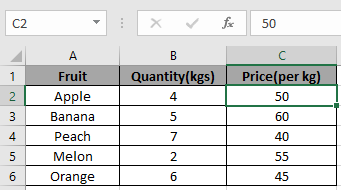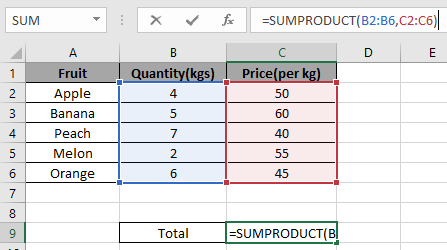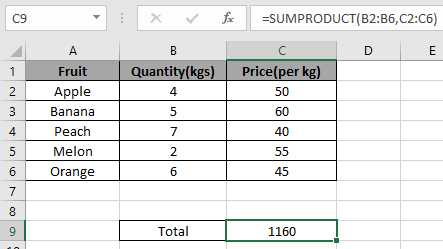# How to Use SUMPRODUCT Function in Excel

SUMPRODUCT function is a mathematical function in Excel. It operates on multiple ranges. It multiplies the corresponding arrays and then adds them.

Total= ( A1 * B1 * C1 * .. + A2 * B2 * C2 * .. + …)

Syntax:

=SUMPRODUCT (array1, [array2], ...)

Let’s understand this by using it in ExampleWe need to find the fruits total amount.

Use the formula to get the total

=SUMPRODUCT(B2:B6,C2:C6)Note: Multiple arrays must-have of an equal number of elements.

You will get the total here.If there’s text in any cell will be considered as 0.

SUMPRODUCT function can be used in counting cells having condition or criteria.

Hope you understood How to use SUMPRODUCT function in Excel. Explore more articles on Totaling arrays here. Please feel free to state your queries regarding this article in the comment box below.

Related Articles:

Get the Rank using SUMPRODUCT function

Count Unique Values using SUMPRODUCT function In Excel

2 Ways to Sum by Month in Excel

Count total matches using SUMPRODUCT in two ranges in Excel

Popular Articles:

How to use the VLOOKUP Function in Excel

How to use the COUNTIF function in Excel 2016

How to Use SUMIF Function in Excel

Terms and Conditions of use

The applications/code on this site are distributed as is and without warranties or liability. In no event shall the owner of the copyrights, or the authors of the applications/code be liable for any loss of profit, any problems or any damage resulting from the use or evaluation of the applications/code.